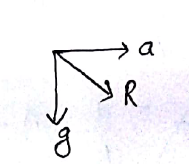# A heavy ball of mass M is suspended from the ceiling of a car by a light string of mass m (m<<M). When the car is at rest, the speed of transverse waves in the string is 60 ms-1. When the car has acceleration a,the wave-speed increases to 60.5 ms-1.The value of a , in terms of gravitational acceleration g, is closest to :Option 1)Option 2)Option 3)Option 4)

Speed of wave on string -

- wherein

Tension in the string

linear mass density

v =

60 =     given m << M

When the car accelerates with aResultant acceleration =

a = 0.1837 g

=

Option 1)

Option 2)

Option 3)

Option 4)

Exams
Articles
Questions